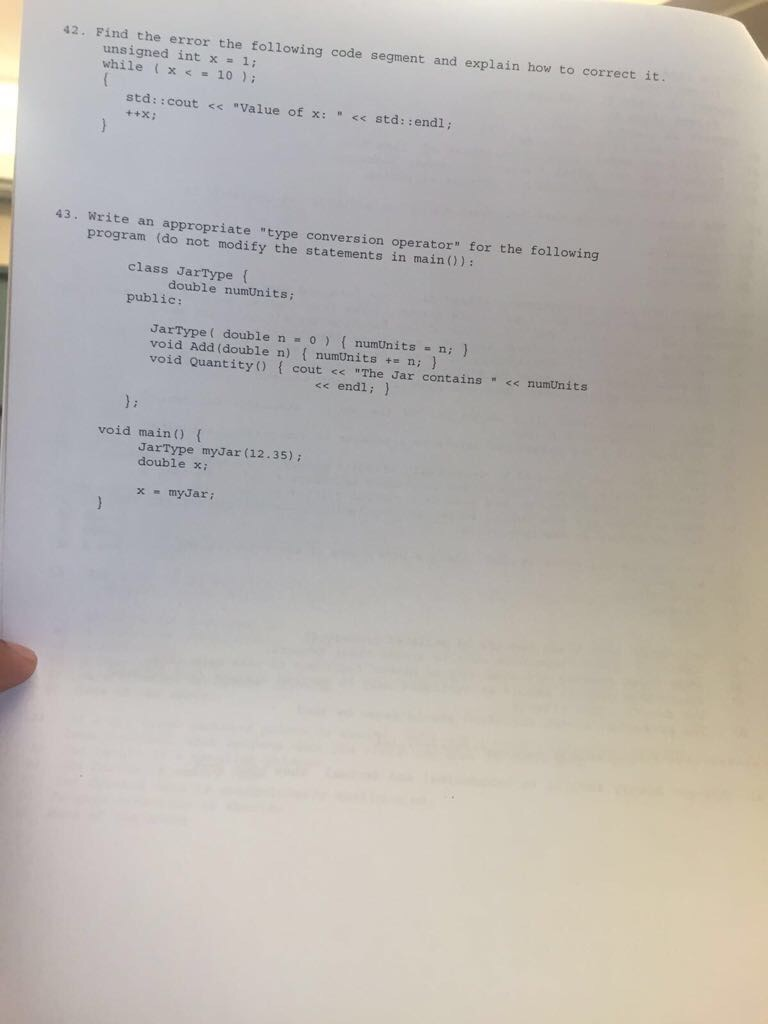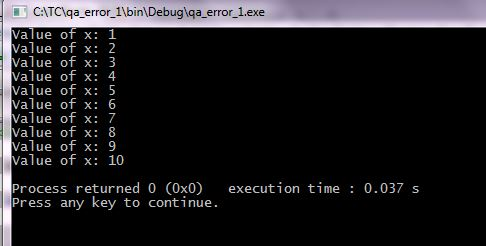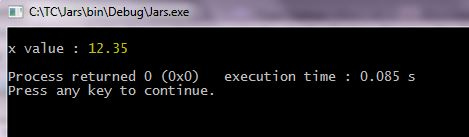# Question & Answer: Find the error the following code segment and explain how to correct it. un…..Find the error the following code segment and explain how to correct it. unsigned int x = 1: while (x

Don't use plagiarized sources. Get Your Custom Essay on
Question & Answer: Find the error the following code segment and explain how to correct it. un…..
GET AN ESSAY WRITTEN FOR YOU FROM AS LOW AS \$13/PAGE

(42)

(1) Value of variable x is initialized to 1.
(2) Semicolon at the end of while loop doesn’t execute the underlying body and continues to execute the while condition until it is satisfied.
(3) Given while condition (x <= 10) will never be true as statements in while body are skipped from execution.
(4) Control goes into infinite loop.

Correction’s Required:

(1) Remove the semicolon after while statement.
(2) Include value of x in output statement.

Updated Program:
#include <iostream>

int main()
{
unsigned int x = 1;

//Remove semicolon
while(x <= 10)

{
std::cout << “Value of x: ” << x << std::endl;

++x;
}

return 0;
}

Sample Run:__________________________________________________________________________________________

(43)

Adding an appropriate type conversion operator:

#include <iostream>

using namespace std;

class JarType{
double numUnits;
public:

JarType( double n = 0 ) { numUnits = n; }
void Add(double n) { numUnits += n; }
void Quantity() { cout << “The Jar contains ” << numUnits << endl; }

//Type Conversion operator for converting JarType object to double
operator double()
{
//Returning double value
return numUnits;
}

};

void main()
{
JarType myJar(12.35);
double x;

x = myJar;

//Printing x value
cout << endl << “x value : ” << x << endl;
}

Sample Run: